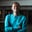Related Tags

php
communitycreator

# How to get the median of an array of numbers in PHPZubair Idris Aweda

The median is the middle value of the given list of data when it is arranged in order. This list can have an even number of elements or an odd number of elements.

In this shot, we will discuss the steps to find the median.

### Step 1: Sort the array

First, we have to sort the array we are going to use. We can use PHP’s built-in sort() method.

For example, let’s sort an array that contains 6, 11, 4, 21, 12.

<?php

// Create an array
$numbers = [6, 11, 4, 21, 12]; // Sort the array sort($numbers);

// Print the sorted array
print_r($numbers); ?> Sorting the array ### Step 2: Get the middle element It’s very straightforward to get the middle element in an array that contains an odd number of elements. You can use the following method to retrieve the index of the middle element: • Get the length of the array • Divide the length by 2 • Round the answer down Now that you have the index of the middle number, the median is the number at that index. See the code below to get the median of a sorted array that contains an odd number of elements. <?php // Create sorted array$numbers = [4, 6, 11, 12, 21];

// Get array length
$length = count($numbers);

// Divide length by 2
$half_length =$length / 2;

// Convert to integer
$median_index = (int)$half_length;

// Get median number
$median =$numbers[$median_index]; // Output median echo$median; // Should be 11

?>
Getting median of an array containing an odd number of elements

In an array with an even number of elements, the process is a bit different, as there’s no middle element. Instead, we have to find the average of the two middle elements.

To get the indices of these two middle elements:

• Get the length of the array
• Divide the length by 2 to get the second middle element
• Subtract 1 from the index of the second middle element to get the first middle element

Now, get the values at these indices and find their average; this value is the median of the array.

Take a look at the code below to find the median of a sorted array that contains an even number of elements.

<?php

// Create sorted array
$numbers = [4, 6, 11, 12, 18, 21]; // Get array length$length = count($numbers); // Divide length by 2$second_half_length = $length / 2; // Subtract 1 from$second_half_length
$first_half_length =$second_half_length - 1;

// Get index values
$first_half =$numbers[$first_half_length];$second_half = $numbers[$second_half_length];

// Get average of these values
$median = ($first_half + $second_half) / 2; // Output median echo$median;

?>
Getting the median of an array containing an even number of elements

RELATED TAGS

php
communitycreator

CONTRIBUTORZubair Idris Aweda
RELATED COURSES

View all Courses

Keep Exploring

Learn in-demand tech skills in half the time